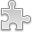# Courses / Module

Toggle Print

##DIFFERENTIAL CALCULUS

Module code: MT101SC
Credits: 5
Semester: 1
Department: MATHEMATICS AND STATISTICS
International:Overview

Objectives: To introduce students to Differential Calculus in One Real Variable.

Overview: Why study calculus?

Sets and Functions. Review of Exponentials, Logarithms and Trigonometry. Inequalities, Limits: Idea of a limit, definition of a limit, continuity. Derivatives: Definition of a derivative, tangents, product rule, chain rule, quotient rule, higher derivatives, implicit differentiation, Newton's method for roots, exponential and logarithmic functions. Applications of derivatives: Maxima and minima, monotonic functions and the first derivative test. Concavity and points of inflection. Symmetry and curve sketching. Applied max-min problems and related rates.Learning OutcomesTeaching & Learning methodsAssessmentAutumn Supplementals/ResitsPre-RequisitesTimetable